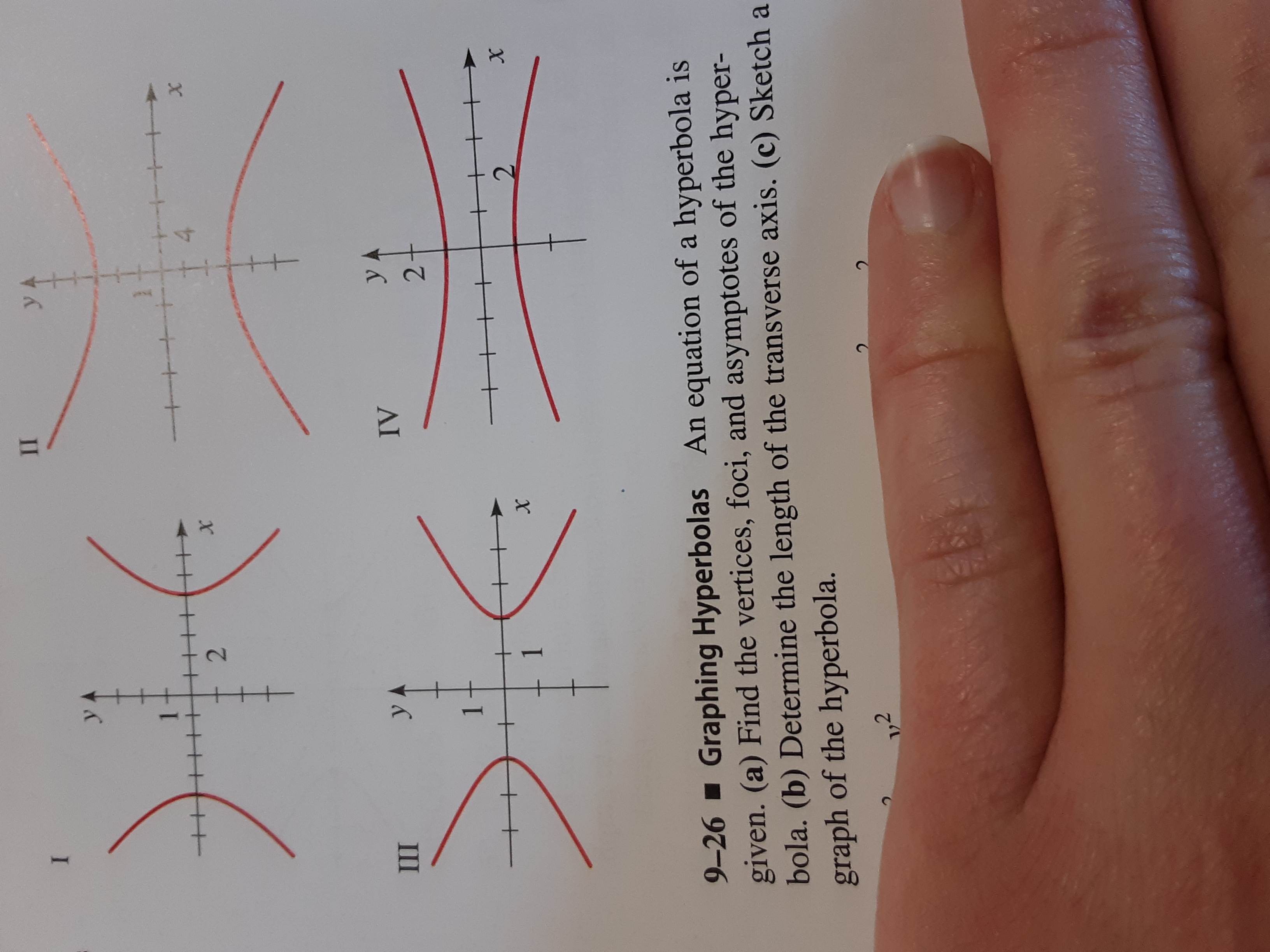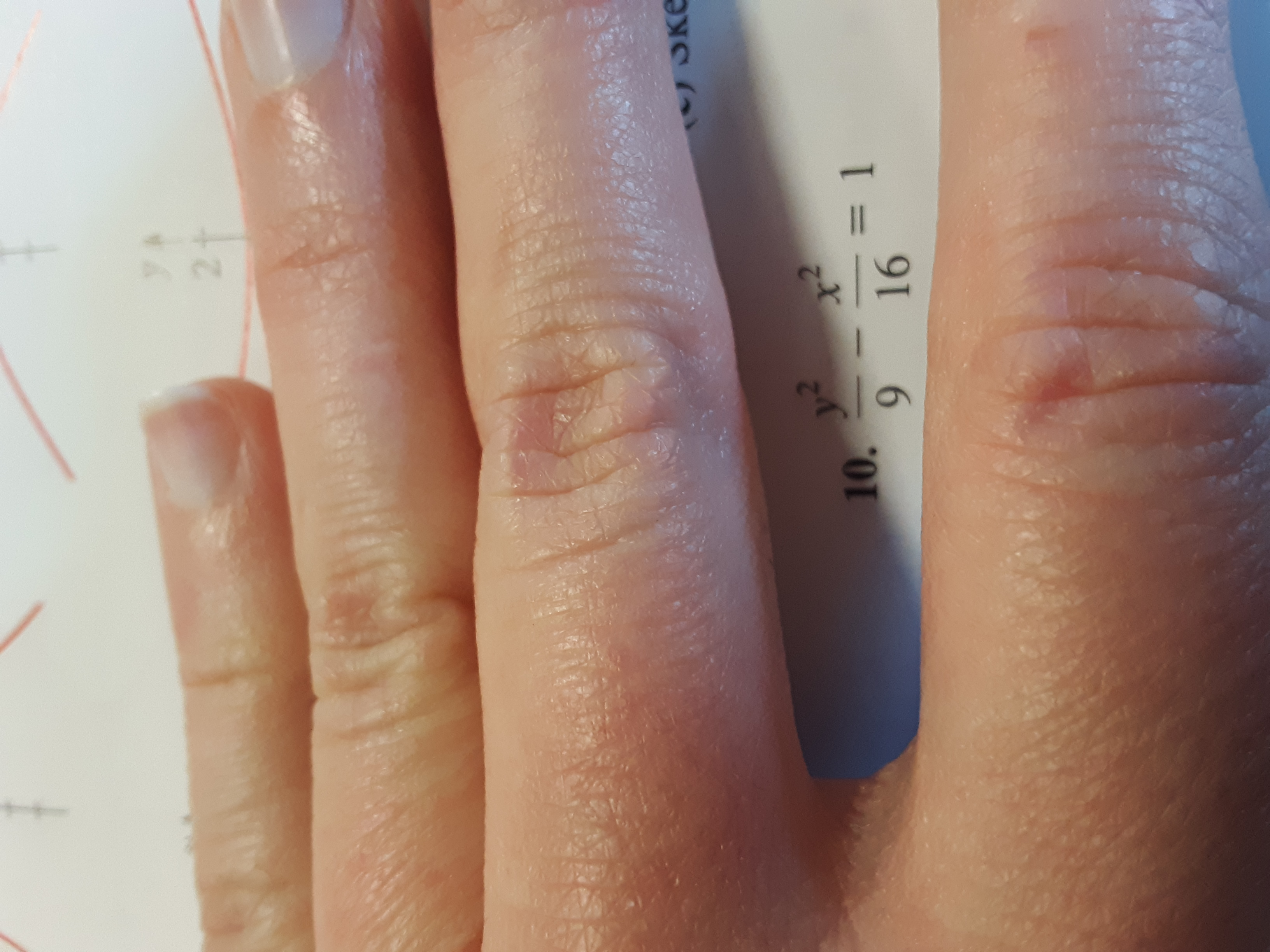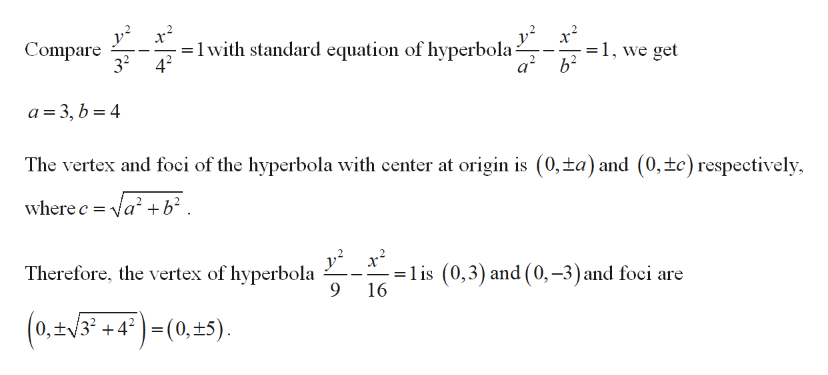# ПIYAу.42XШyAуIV212хх9-26 Graphing Hyperbolas An equation of a hyperbola isgiven. (a) Find the vertices, foci, and asymptotes of the hyper-bola. (b) Determine the length of the transverse axis. (c) Sketch agraph of the hyperbola.1,22+t y A21UOkey210.= 116

Question
15 viewshelp_outlineImage TranscriptioncloseП I YA у. 4 2 X Ш yA у IV 2 1 2 х х 9-26 Graphing Hyperbolas An equation of a hyperbola is given. (a) Find the vertices, foci, and asymptotes of the hyper- bola. (b) Determine the length of the transverse axis. (c) Sketch a graph of the hyperbola. 1,2 2 +t fullscreenhelp_outlineImage Transcriptionclosey A 21 UOke y2 10. = 1 16 fullscreen
check_circle

Step 1

Given,

An equation of a hyperbola is

Step 2

An equation can be written as

Step 3

a)

...help_outlineImage Transcriptionclose=1 with standard equation of hyperbola- a2 1, we get Compare 32 a 3, b 4 The vertex and foci of the hyperbola with center at origin is (0,ta) and (0,tc) respectively where c a +b2 . = lis (0,3) and (0,-3) and foci are 16 Therefore, the vertex of hyperbola 9 (0,#/3 +4°) =(0,+5) fullscreen

### Want to see the full answer?

See Solution

#### Want to see this answer and more?

Solutions are written by subject experts who are available 24/7. Questions are typically answered within 1 hour.*

See Solution
*Response times may vary by subject and question.
Tagged in

### Other